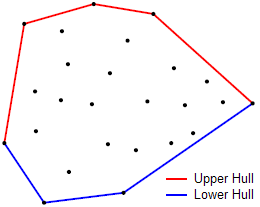Monotone Chain for Convex Hulls

This algorithm finds the convex hull of a set S of points with O(n lg n) time complexity. The convex hull of a geometric object is the smallest (convex) set containing that object. In a bidimensional polygon, one can imagine the convex hull as the format a rubber band would take if it was released and let snap around the points.

The naive approach has O(n3) time complexity because there are O(n2) pairs of points and O(n) comparisons per pair to ensure that all other points lie either to the right or to the left of the segment formed by a given pair. As it is often too slow in practice, I won’t waste more characters about it here.

The O(n lg n) time of this algorithm arises from the need to sort the points. If your input is already sorted, it becomes a O(n) algorithm.

The algorithm builds the convex hull by first making an upper hull and a lower hull and then merging both hulls together.An illustration from www.algorithmist.com.

In this example (where the points are sorted first by nondecreasing values of x, then by nondecreasing values of y), the upper hull starts with the uppermost leftmost point. Iterating to the right, while the partial hull has two or more points and the arc formed by the last two points of the hull and the next point of the ordered set is positively oriented, pop out the last point of the partial hull. This new point should then be added to the hull.

The code for this procedure is given below.

for p in points:
while len(upper) >= 2 and cross(upper[-2], upper[-1], p) >= 0:
upper.pop()
upper.append(p)

cross(o, a, b) here is the cross product of two column vectors (OA and OB). It is a positive value, if OAB makes a counter-clockwise turn, negative for clockwise turn, and zero if the points are collinear.

A similar, but vertically mirrored version of the procedure described above is used to generate the lower hull. In the end, it is a simple matter of combining both hulls into a single one and returning it.

The complete algorithm is as follows.

def cross(a, b, o):
x = (a - o) * (b - o)
y = (a - o) * (b - o)
return x - y

def convex_hull(points):
points = list(sorted(points))
upper = []
for p in points:
while len(upper) >= 2 and cross(upper[-2], upper[-1], p) >= 0:
upper.pop()
upper.append(p)
lower = []
for p in points[::-1]:
while len(lower) >= 2 and cross(lower[-2], lower[-1], p) >= 0:
lower.pop()
lower.append(p)
hull = upper
hull.pop()
hull.extend(lower)
hull.pop()
return hull